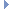OpenMM
LocalCoordinatesSite Class Reference

This is a VirtualSite that uses the locations of three other particles to compute a local coordinate system, then places the virtual site at a fixed location in that coordinate system. More...Inheritance diagram for LocalCoordinatesSite:

List of all members.

## Public Member Functions

def getOriginWeights
getOriginWeights(self) -> Vec3 const &
def getXWeights
getXWeights(self) -> Vec3 const &
def getYWeights
getYWeights(self) -> Vec3 const &
def getLocalPosition
getLocalPosition(self) -> Vec3 const &
def __init__
__init__(self, particle1, particle2, particle3, originWeights, xWeights, yWeights, localPosition) -> LocalCoordinatesSite __init__(self, other) -> LocalCoordinatesSite

this

## Detailed Description

This is a VirtualSite that uses the locations of three other particles to compute a local coordinate system, then places the virtual site at a fixed location in that coordinate system.

The origin of the coordinate system and the directions of its x and y axes are each specified as a weighted sum of the locations of the three particles:

origin = w1r1 + w2r2 + w3r3

xdir = w1r1 + w2r2 + w3r3

ydir = w1r1 + w2r2 + w3r3

For the origin, the three weights must add to one. For example if (w1, w2, w3) = (1.0, 0.0, 0.0), the origin of the local coordinate system is at the location of particle 1. For xdir and ydir, the weights must add to zero. For excample, if (w1, w2, w3) = (-1.0, 0.5, 0.5), the x axis points from particle 1 toward the midpoint between particles 2 and 3.

The z direction is computed as zdir = xdir x ydir. To ensure the axes are all orthogonal, ydir is then recomputed as ydir = zdir x xdir. All three axis vectors are then normalized, and the virtual site location is set to

origin + x*xdir + y*ydir + z*zdir

## Constructor & Destructor Documentation

 def __init__ ( self, args )

__init__(self, particle1, particle2, particle3, originWeights, xWeights, yWeights, localPosition) -> LocalCoordinatesSite __init__(self, other) -> LocalCoordinatesSite

Create a new LocalCoordinatesSite virtual site.

Parameters:
 particle1 (int) the index of the first particle particle2 (int) the index of the second particle particle3 (int) the index of the third particle originWeights (Vec3) the weight factors for the three particles when computing the origin location xWeights (Vec3) the weight factors for the three particles when computing xdir yWeights (Vec3) the weight factors for the three particles when computing ydir localPosition (Vec3) the position of the virtual site in the local coordinate system

## Member Function Documentation

 def getLocalPosition ( self )

getLocalPosition(self) -> Vec3 const &

Get the position of the virtual site in the local coordinate system.

 def getOriginWeights ( self )

getOriginWeights(self) -> Vec3 const &

Get the weight factors for the three particles when computing the origin location.

 def getXWeights ( self )

getXWeights(self) -> Vec3 const &

Get the weight factors for the three particles when computing xdir.

 def getYWeights ( self )

getYWeights(self) -> Vec3 const &

Get the weight factors for the three particles when computing ydir.

## Member Data Documentation

The documentation for this class was generated from the following file:
• openmm.py